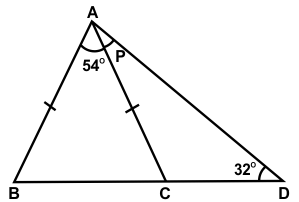﻿ Maths Olympiad (CMO) Previous Year Paper for Class 7

# CREST Mathematics Olympiad Class 7 Previous Year Papers

CREST Mathematics Olympiad Previous Year Paper PDF for Class 7:

## Syllabus:

Section 1: Integers, Fractions and Decimals, Exponents and Powers, Algebraic Expressions, Simple Linear Equations, Lines and Angles, Comparing Quantities, The Triangle and its Properties, Symmetry, Congruence of Triangles, Rational Numbers, Perimeter and Area, Data Handling, Visualizing Solid Shapes, Practical Geometry.

Achievers Section: Higher Order Thinking Questions - Syllabus as per Section 1

 Q.1 Fill in the blank:If two angles and the included side of one triangle are equal to two angles and the included side of the other triangle, then the triangles are congruent as per the _________
 Q.2 If two numbers are in the ratio 2:3 and the product of their HCF and LCM is 33750, then the sum of the numbers is:
 Q.3 Which of the following is a solution of 12x - 5 > 8x + 3?
 Q.4 If 2a = 4b = 16c = 256, then what is the value of 2a + 3b + 4c?
 Q.5 Find the value of ∠x:Q.6 In the figure shown below, ABC is an isosceles triangle and BCD is a straight line. The value of p is:Q.7 An article is listed at \$900 and two successive discounts of 8% and 8% are given on it. How much would the seller gain or lose, if he gives a single discount of 16%, instead of two discounts?
 Q.8 The population of a village increases at the rate 5% p.a. If present population is 8000, then after how many years the population will be 9261?
 Q.9 A laptop is listed at \$1400 and the discount offered is 10%. What additional discount must be given to bring the net selling price to \$1200?
 Q.10 The ratio of the length and breadth of a rectangle is 5:2, respectively. The respective ratio of its perimeter and area is 1:3 (irrespective of the unit). What is the length of the rectangle?

 Q.1 c Q.2 d Q.3 d Q.4 c Q.5 a Q.6 b Q.7 b Q.8 b Q.9 c Q.10 c

Students can download the CREST Mathematics Olympiad previous year paper pdf for class 7 from this page. Each question in the paper carries 1 mark. The answer key for all the questions is also provided on the last page.

The CREST Mathematics Olympiad past year papers for class 7 are a dependable resource to prepare for the CREST Mathematics Olympiad exam. By solving the CREST Mathematics Olympiad previous year paper, students can obtain an idea of the kinds of questions that will be asked in the exam. Also, practicing the previous year paper questions helps students to revise concepts quickly.